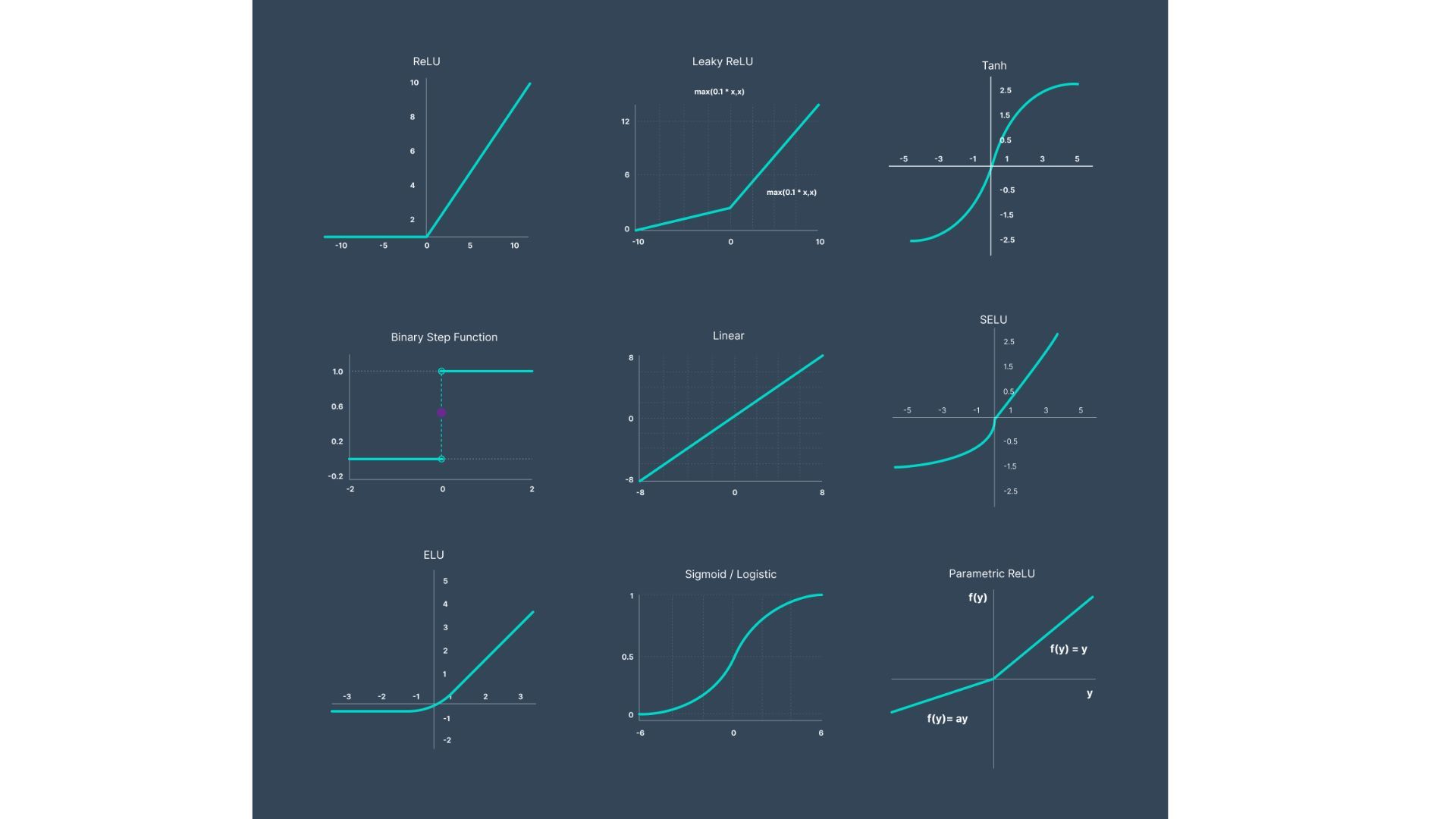# Understanding Activation Function in Neural Network## What is Activation Function?

An activation function is used to determine the result of a neural network. It is also known as the Transfer function. Using the Activation function can help to normalize the input data in any range from 0 to 1 or 1 to -1.

Sometimes a neural network is trained on a million points so the activation function must be really fast so the computation time is less.

The neuron is basically a weighted average of input, then this sum is passed through an activation function to get an output.

``````    Y = Σ(bias + weights * input)
``````

For a neuron Y can vary from -∞ to +∞, so the output needs to be bounded to get the desired prediction and generalized results.

``````    Y = Activation function(Σ(bias + weights * input))
``````

## Need for Activation Functions

In small terms, we can say that activation functions are used to avert linearity. If activation functions won’t use the data would pass through the layers of a network using only linear functions of type `a * x + b`.

The configuration of all these linear functions is again a linear function so even if the data passes through more layers, the yield is always the result of a linear function.

## Types of Activation Functions

An essential neural network is divided into three major parts:

1. Binary Step function
2. Linear function
3. Non-linear activation function

1. Binary step function

The binary step function is the most familiar activation function in neural networks. As the name suggests this function yields a binary output. It produces 0 when the input does not pass the threshold limit whereas produces 1 when the input does pass the threshold limit.

This function is not really accommodating when there are multiple classes to work with. It is mostly used as an activation function while creating a binary classifier.

2. Linear function

The linear function is a simple straight-line function that is directly proportional to the weighted summation of neurons. Linear functions are represented by equation `f(x)=Kx` where K is a constant

The linear function can handle more than one class but does have several disadvantages. For example, the range of linear functions cannot be defined. It has the range from -∞ to ∞. Due to this linear functions cannot work with complex problems.

3. Non-Linear Activation function

The non-linear functions are known to be the most used activation functions. It makes it easy for a neural network model to adapt to a variety of data and to differentiate between the outcomes.
Some of the non-linear activation functions are:

1. Sigmoid Activation Functions
2. Tanh Activation Functions
3. ReLU Activation Functions
4. Maxout
5. ELU
6. Softmax Activation Functions

## When to use which Activation function?

Choosing a particular activation function depends upon the type of problem and in what range is the output desired. For example, to predict values larger than 1 ReLU can be used Tanh and Sigmoid are preferred if the output values have to be in the range (0,1) or (-1, 1).